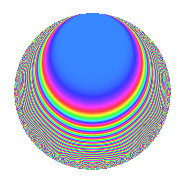# Properties

 Label 1800.1.dgLevel 1800 Weight 1 Character orbit dg Rep. character $$\chi_{1800}(211,\cdot)$$ Character field $$\Q(\zeta_{30})$$ Dimension 16 Newforms 1 Sturm bound 360 Trace bound 0

# Related objects

## Defining parameters

 Level: $$N$$ = $$1800 = 2^{3} \cdot 3^{2} \cdot 5^{2}$$ Weight: $$k$$ = $$1$$ Character orbit: $$[\chi]$$ = 1800.dg (of order $$30$$ and degree $$8$$) Character conductor: $$\operatorname{cond}(\chi)$$ = $$1800$$ Character field: $$\Q(\zeta_{30})$$ Newforms: $$1$$ Sturm bound: $$360$$ Trace bound: $$0$$

## Dimensions

The following table gives the dimensions of various subspaces of $$M_{1}(1800, [\chi])$$.

Total New Old
Modular forms 48 48 0
Cusp forms 16 16 0
Eisenstein series 32 32 0

The following table gives the dimensions of subspaces with specified projective image type.

$$D_n$$ $$A_4$$ $$S_4$$ $$A_5$$
Dimension 0 0 0 16

## Trace form

 $$16q + 4q^{3} - 2q^{4} - 4q^{9} + O(q^{10})$$ $$16q + 4q^{3} - 2q^{4} - 4q^{9} + 4q^{10} + 6q^{11} + 2q^{12} - 2q^{14} + 2q^{16} - 4q^{17} - 4q^{19} - 2q^{25} - 8q^{26} + 4q^{27} + 16q^{30} + 4q^{33} + 4q^{35} - 2q^{36} - 8q^{40} + 4q^{41} + 2q^{42} - 8q^{43} - 8q^{44} - 4q^{46} - 2q^{48} - 16q^{51} + 2q^{56} - 16q^{57} - 4q^{58} - 4q^{62} + 4q^{64} + 4q^{65} + 6q^{67} + 8q^{68} + 2q^{75} + 8q^{76} - 12q^{78} - 4q^{81} + 4q^{83} - 4q^{89} + 4q^{90} - 8q^{91} + 4q^{94} - 4q^{99} + O(q^{100})$$

## Decomposition of $$S_{1}^{\mathrm{new}}(1800, [\chi])$$ into irreducible Hecke orbits

Label Dim. $$A$$ Field Image CM RM Traces $q$-expansion
$$a_2$$ $$a_3$$ $$a_5$$ $$a_7$$
1800.1.dg.a $$16$$ $$0.898$$ $$\Q(\zeta_{60})$$ $$A_{5}$$ None None $$0$$ $$4$$ $$0$$ $$0$$ $$q+\zeta_{60}^{29}q^{2}+\zeta_{60}^{18}q^{3}-\zeta_{60}^{28}q^{4}+\cdots$$